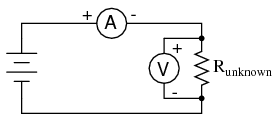# 3.14: 4-wire Resistance Measurement

PARTS AND MATERIALS

• 6-volt battery
• Electromagnet made from experiment in previous chapter, or a large spool of wire

It would be ideal in this experiment to have two meters: one voltmeter and one ammeter. For experimenters on a budget, this may not be possible. Whatever ammeter is used should be capable measuring at least a few amps of current. A 6-volt “lantern” battery essentially short-circuited by a long piece of wire may produce currents of this magnitude, and your ammeter needs to be capable of measuring it without blowing a fuse or sustaining other damage. Make sure the highest current range on the meter is at least 5 amps!

CROSS-REFERENCES

Lessons In Electric Circuits, Volume 1, chapter 8: “DC Metering Circuits”

LEARNING OBJECTIVES

• Operating principle of Kelvin (4-wire) resistance measurement
• How to measure low resistances with common test equipment

SCHEMATIC DIAGRAMILLUSTRATIONINSTRUCTIONS

Although this experiment is best performed with two meters, and indeed is shown as such in the schematic diagram and illustration, one multimeter is sufficient.

Most ohmmeters operate on the principle of applying a small voltage across an unknown resistance (Runknown) and inferring resistance from the amount of current drawn by it. Except in special cases such as the megger, both the voltage and current quantities employed by the meter are quite small.

This presents a problem for measurement of low resistances, as a low resistance specimen may be of much smaller resistance value than the meter circuitry itself. Imagine trying to measure the diameter of a cotton thread with a yardstick, or measuring the weight of a coin with a scale built for weighing freight trucks, and you will appreciate the problem at hand.

One of the many sources of error in measuring small resistances with an ordinary ohmmeter is the resistance of the ohmmeter’s own test leads. Being part of the measurement circuit, the test leads may contain more resistance than the resistance of the test specimen, incurring significant measurement error by their presence:One solution is called the Kelvin, or 4-wire, resistance measurement method. It involves the use of an ammeter and voltmeter, determining specimen resistance by Ohm’s Law calculation. A current is passed through the unknown resistance and measured. The voltage dropped across the resistance is measured by the voltmeter, and resistance calculated using Ohm’s Law (R=E/I). Very small resistances may be measured easily by using large current, providing a more easily measured voltage drop from which to infer resistance than if a small current were used.

Because only the voltage dropped by the unknown resistance is factored into the calculation—not the voltage dropped across the ammeter’s test leads or any other connecting wires carrying the main current—errors otherwise caused by these stray resistances are completely eliminated.

First, select a suitably low resistance specimen to use in this experiment. I suggest the electromagnet coil specified in the last chapter or a spool of wire where both ends may be accessed. Connect a 6-volt battery to this specimen, with an ammeter connected in series. WARNING: the ammeter used should be capable of measuring at least 5 amps of current so that it will not be damaged by the (possibly) high current generated in this near-short circuit condition. If you have a second meter, use it to measure the voltage across the specimen’s connection points, as shown in the illustration, and record both meters’ indications.

If you have only one meter, use it to measure current first, recording its indication as quickly as possible, then immediately opening (breaking) the circuit. Switch the meter to its voltage mode, connect it across the specimen’s connection points, and re-connect the battery, quickly noting the voltage indication. You don’t want to leave the battery connected to the specimen for any longer than necessary for obtaining meter measurements, as it will begin to rapidly discharge due to the high circuit current, thus compromising measurement accuracy when the meter is re-configured and the circuit closed once more for the next measurement. When two meters are used, this is not as significant an issue, because the current and voltage indications may be recorded simultaneously.

Take the voltage measurement and divide it by the current measurement. The quotient will be equal to the specimen’s resistance in ohms.